Published: 30 June 2013

# Optimization of cutting parameters using multi-objective evolutionary algorithm based on decomposition

Fu Tao1
Liu Weijun2
Zhao Jibin3
1University of Chinese Academy of Sciences, China
1, 2, 3Shenyang Institute of Automation, Chinese Academy of Sciences, China
Corresponding Author:
Fu Tao
Views 209

#### Abstract

High-strength die steel is widely used for cutting, stamping and molding because of its advantages of high hardness, high toughness and abrasion resistance. However, its efficiency in cutting has always been difficult. In a complex machining process, the optimization of cutting parameters has a significant influence on the stability and quality of products. In this study, an orthogonal experiment of NAK80 for milling is carried out with cutting speed, feed rate and depth of cut, all of which are considered as relevant variables. According to the experimental results, statistical models for cutting force and surface roughness are built using a regression method as objective functions. Superadded the theoretical formula of material removal rate, a multi-objective evolutionary algorithm based on decomposition is used to optimize the cutting parameters and a Pareto solution set is obtained finally.

## 1. Introduction

As a kind of new high-strength plastic die steel, NAK80 possesses many notable characteristics, such as excellent mirror grinding performance, strong electrical discharge machining performance, higher pre-hardened hardness. It is widely used in manufacturing molds of LCD panels, video cameras, transparent covers, and transparent film. Study of the machinability of this material has a great meaning for ensuring the mold quality, improving processing efficiency and reducing production costs.

Once the processing of materials, cutting tools, cutting methods and process route are determined, the choice of the appropriate cutting parameters has become a very crucial issue, which is considered as the problem of optimization of cutting parameters. Optimization is one of the major problems involved in the engineering application and scientific research. Among them, the problem with only one objective function is known as the single-objective optimization, while that need to be dealt with two or more functions at the same time is called multi-objective optimization problems (MOPs). For Mops, a solution may be better for one goal, but poor for the others. Therefore, a set of compromise solutions may exist, which is known as Pareto-optimal set or non-dominated set. At first, multi-objective optimization problem is often transformed to single objective problem through the weighted method, and then solved by mathematical programming methods. But the optimal solution can be acquired in the case of only one weight. Meanwhile, since the objective and constraint functions may be non-linear, non-differentiable or discontinuous, the traditional mathematical programming methods tend to be less efficient and more sensitive to the weight values or the order given according to the objectives. Evolutionary algorithm can seek in the global scope through maintaining populations by potential solutions between generations. The method from population to population is very useful to search Pareto optimal solution for multi-objective optimization problems.

Since Taylor introduced the pioneering concept of optimal speed into metal processing in 1907 , an increasing number of optimization methods for cutting parameters has been proposed to improve product quality. In recent years, many soft computing techniques, such as fuzzy set theory, neural networks, genetic algorithm, are used to optimize parameters in conventional machining processes, including turning, milling, drilling and grinding. Nandi and Pratihar developed an expert system based on a fuzzy basis function network for predicting surface roughness in ultra-precision turning . The parameters of the network were optimized with a genetic algorithm code. Chen and Savage developed a model using a neural fuzzy system to predict surface roughness under different combinations of tool and work piece for end milling process, and a 90 % accuracy of prediction average can be obtained .

Quiza et al. carried out an experimental investigation on tool wear prediction by using ceramic cutting tools for turning hardened cold rolled tool steel . The neural network and regression models were compared in predicting tool wear for different values of cutting speed, feed and time and they found the neural network had shown better capability to make accurate predictions. Khanchustambham and Zhang used neural network to predict cutting force as well as surface finish during machining of advanced ceramic material . Parameters such as spindle speed, feed and depth of cut are employed, and the network is trained by cutting force signal and measured surface finish for online monitoring of the turning process.

Apart from the above techniques, genetic algorithm has been used for optimizing cutting parameters in surface roughness and other performances in particular. Machining parameters such as number of passes, depth of cut during each pass, speed and feed are obtained using a genetic algorithm to optimize minimum production cost in face milling operation by Shunmugam et al. . Authors found that the proposed optimization method yields better results than those reported in the existing literature. Sreeram et al. also used genetic algorithms to optimize cutting parameters for maximizing tool life and minimizing production cost in micro end milling operations . Depth of cut, considered as one of the critical variables along with cutting speed and feed rate has been treated as input parameters. Besides, the authors found more improved results compared to tool manufacturers. Datta et al. presented a study wherein optimizing the cutting speed, feed and depth of cut for a turning process, while production time, production cost and surface roughness are considered as objectives . The multi-objective genetic algorithm NSGA-II  is used to get a Pareto-optimal front to solve the three-objective optimization problem and an innovization study is carried out to find relationship that variable values may exist among obtained points. Chen proposed an improved NSGA-II by extending the non-dominance concept to constraint space in order to deal with multiple constraints in a milling process, in which minimal machining time and minimal elapsed tool life were considered . The authors found that the algorithm makes the optimization process more approximate to application, more economical and effective in searching the Pareto front.

In addition, other soft computing tools are applied for machining process optimization. Lee et al. developed an abductive network for modeling drilling processes with tool life, metal removal rate, thrust force and torque as performances, while cutting speed, feed rate and drill diameter as input parameters , then a simulated annealing optimization was applied to search for optimal parameters considering productivity and tool cost. Ghaiebi and Solimanpur developed an ant algorithm to minimize the summation of tool travel and tool switch times in multiple hole-making operations employing several tools, and the proposed method is proved effectively and efficiently by the computational experience . Prakasvudhisarn et al. proposed a combined approach to determine optimal cutting conditions for desired surface roughness in end milling . The approach consists of two parts: a machine learning technique called support vector machines (SVMs) to predict surface roughness and particle swarm optimization (PSO) technique to optimize process parameters. Tendon et al. used an artificial neural network (ANN) to predict the cutting forces that in turn was used to optimize both feed and speed with particle swarm optimization algorithm for NC pocket milling process. They observed that the approach reduces machining time up to 35 % .

The following paper focuses on a multi-objective evolutionary algorithm based on decomposition (MOEA/D) to get Pareto optimizing solution of cutting parameters in the end milling process of high-strength die steel NAK80. Statistical models for cutting force and surface roughness will be built with parameters of cutting speed, feed rate and cutting depth based on the Taguchi’s orthogonal experiment. Adding the theoretical formula of material removal rate as the objective functions, the MOEA/D method based on Pareto optimal solution sets is applied to cutting parameters optimization. A group of optimum combination of cutting parameters conformed to requirements is obtained.

## 2. Experimental procedure

### 2.1. Workpiece and tool materials

(1) The selected work material in this study is NAK80, the hardness of which is HB 344-400. Both the chemical composition and physical and mechanical properties are shown in Table 1 and Table 2 respectively.

Table 1Chemical composition of NAK80

 Element C Si Ni Mn Mo Cu Al Cr Content 0.15 0.3 3.0 1.5 0.3 1.0 1.0 0.3

Table 2Physical and mechanical properties of NAK80

 Material Temperature$T$ / ºC Tensile strength${\sigma }_{b}$ / MPa Elongation$\delta$ / % Contraction$\Psi$ / % Yield${\sigma }_{s}$ / MPa Modulus of elasticity$E$ / GPa NAK80 25 1319 14.6 51.2 1186 199

(2) The experimental study is carried out on a high-speed machining center VMC0745. Without any cutting fluid, the experiment is conducted in the style of down milling, as shown in Fig. 1. 9433 bench is used in the dynamometer, and cutting force acts on the Kilstler 9265B piezoelectric sensors to produce the charge signal, which can be amplified by multi-channel charge amplifier 5070. These signals are collected through data acquisition card and transmitted to the computer. The software of data processing (DynoWare) is used to test and analyze the collected signals. The surface roughness can be measured by MarSurf PS1, which is produced in Germany as a portable roughness measuring instrument.

Fig. 1Milling experiment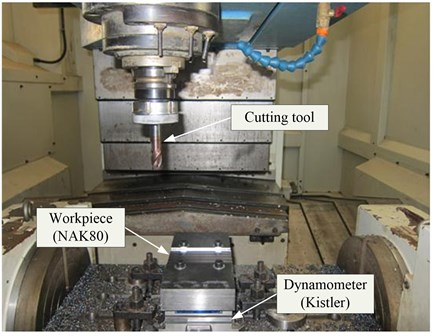(3) The cutting tools are made of solid carbide and coated with TiSiN under major specifications, such as diameter of 16 mm ($\Phi$), teeth of 4, helix angle of 35, and the $R$ angle of 1.

### 2.2. Experimental design

The experiment is designed using the Taguchi method, which focuses on minimizing process variation or follows the least sensitive manner towards the environmental variation for products and process. The Taguchi method can also realize the robust and optimal operation under the varied environment conditions with high efficiency. Due to the material cutting principle, major influential factors are cutting speed ($v$), feed rate ($f$) and depth of cut ($d$). The width of cut ($w$) is fixed to 10 mm. The three factors are employed as cutting parameters to build experimental tables, and each factor is set three levels, shown in Table 3. The three factors with every three levels consist of L27 orthogonal array, shown in Table 4. The cutting force ($F$), surface roughness ($Ra$) and material removal rate ($MRR$) are engaged as responses.

Table 3Cutting parameters and their levels

 Level Cutting parameters Cutting speed$v$ / m·min-1 Feed rate$f$ / mm·rev-1 Depth of cut$d$ / mm 1 100 0.40 0.20 2 120 0.56 0.35 3 140 0.72 0.50

Table 4Experimental array

 Order Cutting parameters Responses Cuttingspeed$v$ / m·min-1 Feedrate$f$ / mm·rev-1 Depthof cut$d$ / mm Cuttingforce$F$ / N Surfaceroughness$Ra$ / μm Materialremoval rate$MRR$ / mm3·min-1 1 100 0.40 0.20 114.60 0.523 1591.55 2 100 0.40 0.35 157.50 0.848 2785.21 3 100 0.40 0.50 196.60 1.204 3978.87 4 100 0.56 0.20 116.20 0.588 2228.17 5 100 0.56 0.35 175.70 0.909 3899.30 6 100 0.56 0.50 233.40 1.311 5570.42 7 100 0.72 0.20 121.30 0.667 2864.79 8 100 0.72 0.35 207.10 1.022 5013.38 9 100 0.72 0.50 288.10 1.482 7161.97 10 120 0.40 0.20 98.41 0.485 1909.86 11 120 0.40 0.35 165.60 0.821 3342.25 12 120 0.40 0.50 246.40 1.035 4774.65 13 120 0.56 0.20 90.33 0.544 2673.80 14 120 0.56 0.35 176.60 0.859 4679.16 15 120 0.56 0.50 245.30 1.180 6684.51 16 120 0.72 0.20 106.00 0.618 3437.75 17 120 0.72 0.35 225.90 0.895 6016.06 18 120 0.72 0.50 325.30 1.354 8594.37 19 140 0.40 0.20 94.65 0.467 2228.17 20 140 0.40 0.35 173.40 0.806 3899.30 21 140 0.40 0.50 260.60 0.954 5570.42 22 140 0.56 0.20 101.30 0.489 3119.44 23 140 0.56 0.35 214.80 0.774 5459.01 24 140 0.56 0.50 311.20 1.016 7798.59 25 140 0.72 0.20 121.60 0.564 4010.70 26 140 0.72 0.35 232.50 0.858 7018.73 27 140 0.72 0.50 329.60 1.029 10026.76

## 3. Statistical model

Regression is a technique for investigating functional relationship between input and output of a process, which is suitable for data description for manufacturing process and parameter estimation. In this study, the input includes cutting speed, feed rate and depth of cut, and the output is cutting forces or surface roughness; the relationship between input and output can be modeled based on second-order polynomial equation as given in the following:

$Y={\beta }_{0}+\sum _{i=1}^{k}{\beta }_{i}{X}_{i}+\sum _{i

Material removal rate is another important criterion for determining the machining operation because a higher rate is always preferred in such operations. The theoretical formula of material removal rate with the unit of mm3/min can be calculated using the following expression:

1
$MRR=\frac{1000vfdw}{\pi \Phi }.$

### 3.1. Cutting force

Cutting force is one of the main physical phenomena in the metal-cutting process, which directly affects the cutting heat, tool wear, tool life and the quality of machined surface. Moreover, it can cause vibration, or even damage tools and machine parts. It is also essential in the design of machine tools, cutting tools and fixtures. Especially with the development of automated processing, cutting force is often used as a significant parameter in the adaptive control of cutting process and has been widely appreciated. Therefore, it is not only an important aspect of the cutting mechanism, but also significant for the application. Its statistical model based on regression method is developed by making use of the experimental results and given below:

2
$F=486.636-4.603v-436.691f-467.198d+0.189v×f+6.051v×d+688.056f×d+0.0126{v}^{2}+294.054{f}^{2}-141.506{d}^{2}.$

Table 5Analysis of variance for cutting force

 Source Sum of squares DF Mean square $F$-value Prob. Cont. % Remarks Model 1.4233e+05 9 15814 127.32 <0.0001 – Significant $v$ 2917.2 1 2917.2 23.487 0.0002 2.020 Significant $f$ 11232 1 11232 90.43 <0.0001 7.776 Significant $d$ 1.2039e+05 1 1.2039e+05 969.31 <0.0001 83.353 Significant $v×f$ 4.3802 1 4.3802 0.035265 0.85326 0.003 Not significant $v×d$ 3954.9 1 3954.9 31.841 <0.0001 2.738 Significant $f×d$ 3272.3 1 3272.3 26.345 <0.0001 2.266 Significant ${v}^{2}$ 151.57 1 151.57 1.2203 0.28469 0.105 Not significant ${f}^{2}$ 340 1 340 2.7374 0.11637 0.235 Not significant ${d}^{2}$ 60.823 1 60.823 0.48969 0.49353 0.042 Not significant Error 2111.5 17 124.21 – – 1.462 – Total 1.4444e+05 26 – – – 100 –

The ${R}^{2}$ value of the second-order polynomial model for cutting force is 97.8 %, it can be noted that this model is highly significant at a 95 % confidence level. It provides an excellent relationship between the response and the experimental values. A variance analysis of the model is shown in Table 5. It shows that all the cutting parameters and two-level interaction effect of cutting speed and depth of cut ($v×d$), feed rate and depth of cut ($f×d$) affect on the cutting force. The most significant factor associated with the cutting force is depth of cut, with 83.353 % contribution to the model. The residuals plot, including normal probability plot and residual versus fitted values for cutting force are presented in Fig. 2. It can be seen from Fig. 2a that the residuals fall on a straight line, which means that the errors between the experimental and predicted values are normally distributed, and the regression model is well fitted. Fig. 2b shows that the maximum variation is within the range from –20 to 20, which indicates the existence of high correlation between observed and fitted values.

Fig. 2Residuals plot for cutting force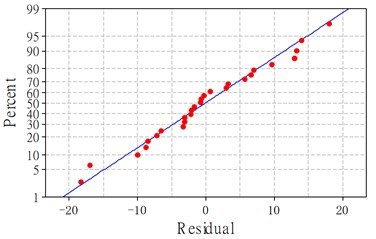a) Normal probability plot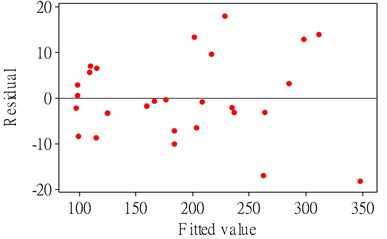b) Residual vs fitted values

### 3.2. Surface roughness

Surface roughness is one of the most important criteria for machining quality measurement. The performance and durability of mechanical parts are not only connected with the material and heat treatment, but also closely related to its surface micro-geometry or surface roughness. The surface roughness directly affects the wear resistance, corrosion resistance, fatigue strength and coordination properties of the mechanical parts:

3
$Ra=-0.8775+0.01055v+0.558f+4.0966d-0.009688v×f-0.020556v×d+1.0347f×d-0.00000986{v}^{2}+0.6337{f}^{2}-0.1827{d}^{2}.$

Table 6Analysis of variance for surface roughness

 Source Sum of squares DF Mean square $F$-value Prob. Cont. % Remarks Model 2.0634 9 0.2293 133.46 <0.0001 – Significant $v$ 0.1417 1 0.1417 82.4836 <0.0001 6.771 Significant $f$ 0.1007 1 0.1007 58.5933 <0.0001 4.810 Significant $d$ 1.7547 1 1.7547 1.0215e+03 <0.0001 83.852 Significant $v×f$ 0.0115 1 0.0115 6.7133 0.0190 0.551 Not significant $v×d$ 0.0456 1 0.0456 26.5652 <0.0001 2.181 Significant $f×d$ 0.0074 1 0.0074 4.3081 0.0534 0.354 Not significant ${v}^{2}$ 9.3352e-05 1 9.3352e-05 0.0543 0.8185 0.0045 Not significant ${f}^{2}$ 0.0016 1 0.0016 0.9192 0.3511 0.076 Not significant ${d}^{2}$ 1.0141e-04 1 1.0141e-04 0.0590 0.8109 0.0049 Not significant Error 0.0292 17 0.0017 – – 1.396 – Total 2.0926 26 – – – 100 –

The model developed above establishes the relationship between the cutting parameters and the response of surface roughness in down milling of NAK80. Similarly to the cutting force, the ${R}^{2}$ value of second-order polynomial regression model for surface roughness is over 98 %, which means that the statistical model reflects the relationship between the cutting parameters and the response. The analysis of variance table of the statistical model for $Ra$ is presented in Table 6, which indicates that the model is highly significant at a 95 % confidence level for that the probability is less than 0.05. Fig. 3a displays the normal probability of residuals for $Ra$, which indicates that the model is well suited for the residuals that are distributed normally and falls in a straight line. The fitted value versus the residuals are shown in Fig. 3b, which shows a high correlation that exists among the experimental values, the model for the residuals is within the range from –0.08 to 0.08 as can be observed.

The comparison between the predicted and measured values for cutting force and surface roughness is shown in Fig. 4. Through the analysis and comparison, it indicates that the two statistical models both have a high accuracy and can be used for the optimization instead of the actual model.

Fig. 3Residuals plot for surface roughness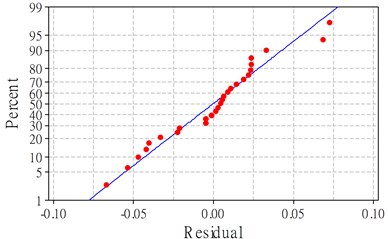a) Normal probability plot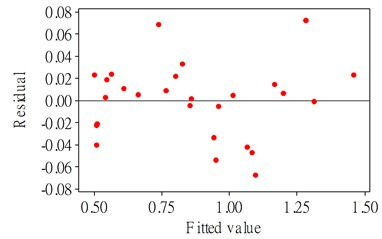b) Residual vs fitted values

Fig. 4Comparison between predicted and measured values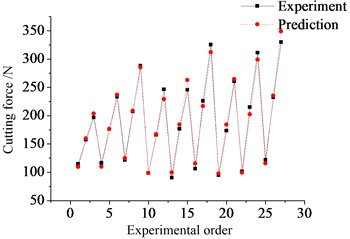a) Cutting force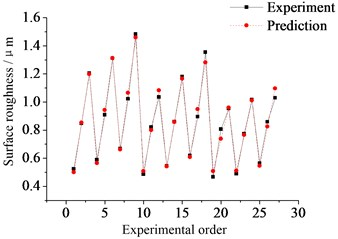b) Surface roughness

## 4. Effect of cutting parameters on responses

According to the significance in the variance analysis of regression models, a further explanation is made for the influence of cutting parameters.

### 4.1. Cutting force

Table 5 shows that cutting speed ($v$), feed rate ($f$), depth of cut ($d$), two-level interaction effect of cutting speed and depth of cut ($v×d$), feed rate and depth of cut ($f×d$) all have significant effect on the cutting force. In Fig. 5a it can be seen that the lowest cutting force can be attained with the combination of the highest cutting speed and smallest depth of cut. The effect of feed rate and depth of cut on cutting force is depicted in Fig. 5b. In the two figures it can be found that along a fixed depth of cut, the cutting force does not change obviously with the increase of cutting speed or feed rate. In other words, the influence of depth of cut is the largest, which can also be determined from the analysis of variance above (Cont. ≈ 83.353 %). The other two critical factors are cutting speed and feed rate with contributions 2.02 % and 7.776 % respectively. Among two-level interaction effects, only the interaction of cutting speed and feed rate has a very weak significance with 0.003 % contribution. The variation of cutting force with each of the cutting parameters in a given range is shown in Fig. 5c, which indicates that the cutting force is growing with the increase of each single factor, but has only a small change for cutting speed.

Fig. 5Effect of cutting parameters on cutting force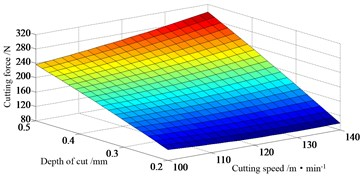a) Interaction effect of cutting speed and depth of cut ($f=$ 0.56 mm·rev-1)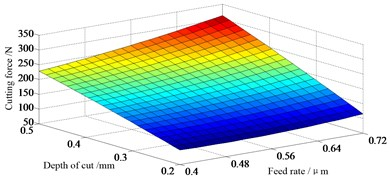b) Interaction effect of feed rate and depth of cut ($v=$ 120 m·min-1)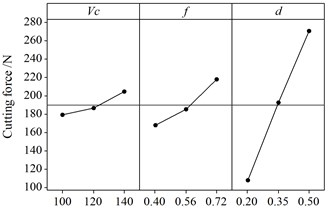c) Main effect of cutting parameters

### 4.2. Surface roughness

Concerning surface roughness, the analysis of variance is summed up in Table 6. Fig. 6a shows the experimental response surface for $Ra$ in relation to cutting speed and depth of cut, while the feed rate is kept at the middle level. The lowest surface roughness is achieved at the highest cutting speed and smallest depth of cut. And it is depicted that the higher the cutting speed or the smaller the depth of cut, the lower the surface roughness will be. This regular pattern can also be found from Fig. 6b. Like cutting force, the factor ($d$) affects surface roughness in a considerable way and the contribution of three cutting parameters is over 93 %. However, the effect of feed rate on the surface roughness is larger than cutting speed while the situation is different on the cutting force. Besides the interaction of cutting speed and depth of cut ($v×d$), other factors do not present any statistical significance on the surface roughness.

Fig. 6Effect of cutting parameters on surface roughness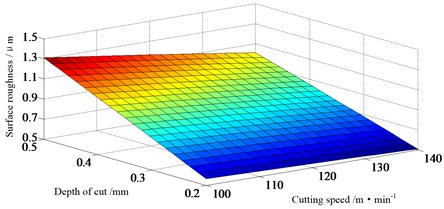a) Interaction effect of cutting speed and depth of cut ($f=$ 0.56 mm·rev-1)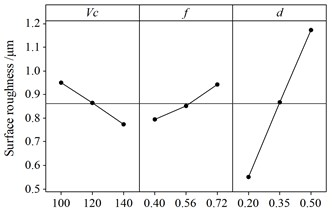b) Main effect of cutting parameters

## 5. Multi-objective optimization

### 5.1. Multi-objective evolutionary algorithm based on decomposition

A multi-objective optimization problem for engineering application and scientific research can be expressed as:

$\mathrm{m}\mathrm{i}\mathrm{n}F\left(x\right)={\left({f}_{1}\left(x\right),\cdots ,{f}_{m}\left(x\right)\right)}^{T},\mathrm{}\mathrm{}\mathrm{}\mathrm{s}\mathrm{u}\mathrm{b}\mathrm{j}\mathrm{e}\mathrm{c}\mathrm{t}\mathrm{}\mathrm{t}\mathrm{o}\mathrm{}x\in \Omega ,$

where $\Omega$ dominates the feasible region of parameters, and $F:\Omega \to {R}^{m}$ consists of $m$ real-valued functions with the space of objective function ${R}^{m}$.

The method of MOEA/D was introduced by Zhang in 2007 , combining the traditional mathematical programming with the evolutionary algorithm. In his paper, the MOP is decomposed into $N$ single objective scalar optimization sub-problems, and each sub-problem is solved by evolutionary algorithm. The population of the evolutionary algorithm is generated from the current optimal solutions of each optimization sub-problem. Then the neighborhood of each pairwise sub-problem is defined as the distance of the couple weights, and the optimization of each sub-problem can be solved by means of evolving manner among the neighbor sub-problems.

Let ${\lambda }^{1},\cdots ,{\lambda }^{N}$ be a group of weight vectors distributing uniformly, ${z}^{*}$ as the reference point. Through the Tchebycheff method, the MOP problem can be decomposed into $N$ scalar optimization problems, in which the objective function of the $j$-th sub-problem is:

${g}^{te}\left(x|{\lambda }^{j},{z}^{\mathrm{*}}\right)=\underset{1\le i\le m}{\mathrm{m}\mathrm{i}\mathrm{n}}\left\{{\lambda }_{i}^{j}\left|{f}_{i}\left(x\right)-{z}_{i}^{\mathrm{*}}\right|\right\},$

where ${\lambda }^{j}={\left({\lambda }_{1}^{j},{\lambda }_{2}^{j},\cdots ,{\lambda }_{m}^{j}\right)}^{T}$ and ${g}^{te}$ is continuous with respect to $\lambda$. The optimal solution of ${g}^{te}\left(x|{\lambda }^{i},{z}^{*}\right)$ should be close to that of ${g}^{te}\left(x|{\lambda }^{j},{z}^{*}\right)$ while ${\lambda }^{i}$ is close to ${\lambda }^{j}$. It means that any information close to ${\lambda }^{i}$ about ${g}^{te}$ will contribute to ${g}^{te}\left(x|{\lambda }^{i},{z}^{*}\right)$.

In MOEA/D, the neighbor of weight vector ${\lambda }^{i}$ is defined as the nearest neighbors of its surround weight vectors $\left\{{\lambda }^{1},\cdots ,{\lambda }^{N}\right\}$. Therefore, the neighbor of the $i$ sub-problem consists of sub-problems which are the nearest neighbor weight vectors to ${\lambda }^{i}$. The optimization of each sub-problem could only be solved by its own surrounding neighbors. In every evolved generation, MOEA/D has the following parameters: 1) the population with size $N:{x}_{1},\cdots ,{x}_{N}\in \Omega$, where ${x}_{i}$ is the current solution of the $i$-th sub-problem, 2) $z={\left({z}_{1},\cdots ,{z}_{m}\right)}^{T}$, where ${z}_{i}$ is the best value of ${f}_{i}$, 3) archive set $A$ is used to store the non-dominated solutions during the optimal process. Stated procedurally, the step of MOEA/D is as follows.

(1) Initialization

• Set the number of evolution iterations as ${t}^{\mathrm{m}\mathrm{a}\mathrm{x}}$, the number of sub-problems as $N$, weight vectors as ${\lambda }^{1},\cdots ,{\lambda }^{N}$, and the number of neighbor weight vectors for each vector as $T$.

• Let $A=\varphi$. Calculate the distance between every two weight vectors and get the $T$ neighbor weight vectors for ${\lambda }^{i}$.

• Let $B\left(i\right)=\left\{{i}_{1},\cdots ,{i}_{T}\right\}$ ($i=1,\cdots ,N$), where ${\lambda }^{{i}_{1}},\cdots ,{\lambda }^{{i}_{T}}$ are the neighbor weight vectors for ${\lambda }^{i}$.

• Initialize the population ${x}_{1},\cdots ,{x}_{N}\in \Omega$ randomly, calculate $F\left({x}_{i}\right)$ and then initialize $z={\left({z}_{1},\cdots ,{z}_{m}\right)}^{T}$.

(2) Iterative calculation

Run the following operations for $i=1,\cdots ,N$:

• Recombination: individuals ${x}_{k}$ and ${x}_{i}$ are randomly selected from $B\left(i\right)$ and then recombined to form a new individual $y$.

• Mutation: $y$ is randomly mutated to form ${y}^{\text{'}}$.

• Update $z:{z}_{j}={f}_{j}\left({y}^{\text{'}}\right)$ if ${z}_{j}<{f}_{j}\left({y}^{\text{'}}\right)$ ($j=1,\cdots ,m$).

• Update the neighbor individuals: ${x}^{j}={y}^{\text{'}}$ and $F\left({x}^{j}\right)=F\left({y}^{\text{'}}\right)$ if ${g}^{te}\left({y}^{\text{'}}|{\lambda }^{j},z\right)\le {g}^{te}\left({x}^{j}|{\lambda }^{j},z\right)$, where $j\in B\left(i\right)$.

• Update archive set $A:$ delete the vectors in $A$ dominated by $F\left({y}^{\text{'}}\right)$, and then add $F\left({y}^{\text{'}}\right)$ to $A$.

• Termination condition

If the termination condition is satisfied, output the archive set $A$. Otherwise, back to (2).

### 5.2. Results and discussion

Based on the above algorithm, MOEA/D is performed to optimize the cutting parameters for the purpose of convergence to the real Pareto boundary. The solutions should have diversity and uniformity and cover the entire boundaries as possible. There is not only a global optimal solution existing in the multi-objective optimization, but also solution sets. The objective functions, derived from equations (2) and (3) and the theoretical formula (1) of material removal rate, can be expressed as follows:

$\begin{array}{c}Min:F=486.636-4.603v-436.691f-467.198d+0.189v×f+6.051v×d\\ +688.056f×d+0.0126{v}^{2}+294.054{f}^{2}-141.506{d}^{2},\end{array}$
$\begin{array}{c}Min:Ra=-0.8775+0.01055v+0.558f+4.0966d-0.009688v×f-0.020556v×d\\ +1.0347f×d-0.00000986{v}^{2}+0.6337{f}^{2}-0.1827{d}^{2},\end{array}$
$Max:M=\frac{1000vfdw}{\pi \Phi }.$

The initialized parameters of MOEA/D are: evolution generations 1000, population size 100, neighbor numbers 20 and decomposition method of Tchebycheff. The final solution sets, composing the Pareto front shown in Fig. 7, are obtained over the entire optimization and 10 out of them are presented in Table 7. Here any solution in the final set is not persuasively better than others, for each one of them can be regarded as the optimal solution under the specific product requirement. Therefore the process engineer should select the optimal solution reasonably. A suitable combination of cutting parameters can be selected from Table 7 if the engineer desires to have a smaller cutting force, a better surface finish or a higher material removal rate.

Fig. 7Pareto optimal front using MOEA/D for three objectives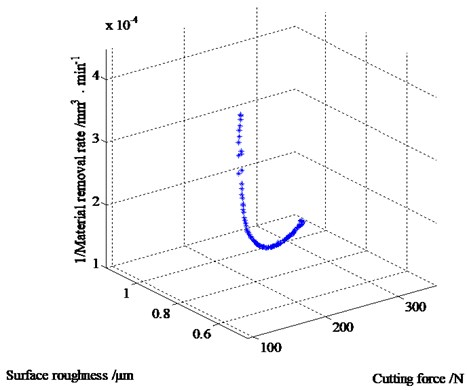From the experimental results presented in Table 4, the responses of order 1 obtained are as follows: $F$ (114.6 N), $Ra$ (0.523 μm) and $MRR$ (1591.55 mm3/min). After optimization of cutting parameters using MOEA/D, it can be seen that $F$ and $Ra$ has been respectively reduced to 109.12 N and 0.5 μm for the approximately same material removal rate (order Table 7).

The varied results obtained from MOEA/D for the responses of cutting force, surface roughness and material removal rate with respect to different cutting parameters are presented in Fig. 8. According to the figure, it can be asserted that most of the Pareto optimal points are concentrated on higher cutting speed, higher feed rate and at a lower depth of cut. Therefore, it can be used as the basis to combine the cutting parameters by engineers.

Table 7Optimal solutions of cutting parameters

 Order Cuttingspeed$v$ / m·min-1 Feedrate$f$ / mm·rev-1 Depthof cut$d$ / mm Cuttingforce$F$ / N Surfaceroughness$Ra$ / μm Materialremoval rate$MRR$ / mm3·min-1 1 100.02 0.40 0.20 109.22 0.500 1591.87 2 100.14 0.40 0.20 109.12 0.500 1593.78 3 139.97 0.72 0.50 348.48 1.096 10024.61 4 139.99 0.69 0.20 111.40 0.536 3843.32 5 140.00 0.72 0.31 204.00 0.751 6216.59 6 140.00 0.72 0.29 188.20 0.714 5815.52 7 140.00 0.41 0.20 97.16 0.507 2283.87 8 139.98 0.46 0.20 96.32 0.505 2562.03 9 140.00 0.69 0.20 111.40 0.536 3843.59 10 140.00 0.52 0.20 97.25 0.507 2896.62

Fig. 8Pareto optimal solutions with respect to individual cutting parameters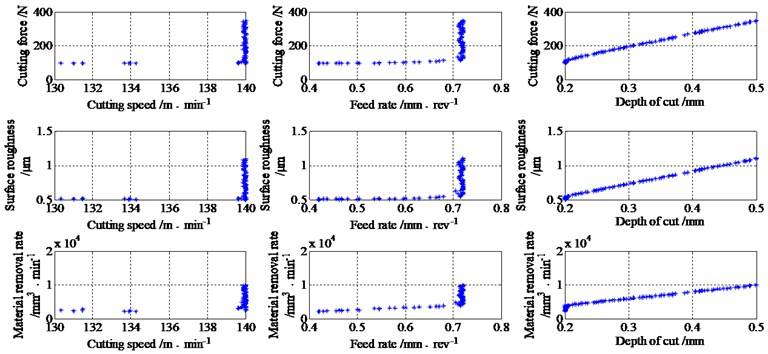## 6. Conclusion

(1) With materials of high-strength die steel NAK80 and a solid carbide tool coated with TiSiN as cutting tool for experiment, the responses of cutting force, surface roughness and material removal rate were acquired.

(2) The statistical models for cutting force and surface roughness were developed by investigating the influences of cutting parameters. An analysis of variance indicates that depth of cut has the most significant influence. Following the theoretical formula of the material removal rate, the objective functions were established for the subsequent optimization of cutting parameters.

(3) A multi-objective evolutionary algorithm based on decomposition was used to obtain the Pareto optimal solution sets of cutting parameters in the milling process of NAK80. Accordingly, a suitable combination of cutting parameters can be selected by engineers.

(4) The method used in this research has a wide range of applications and more cutting parameters can be considered, such as the concentration of cutting fluid, tool parameters and width of cut. Furthermore, other responses, such as tool wear, machine power and so on, can be extended in order to optimize the cutting process and to guide the actual production.